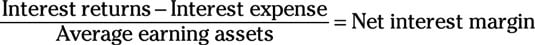##### Corporate Finance For DummiesAnalysts use the net interest margin to determine whether the earning assets are actually making enough money to justify the interest expense or if the company would’ve been better off just paying off its debts to decrease the interest expense.

In other words, a good way to determine whether a company is effectively using its earning assets is to look at the proportion of income that’s being generated for the value of the company’s assets.

To find out, analysts use the net interest margin:Use the following steps to work through this equation:

1. Find the interest returns and interest expense on the income statement.

2. Use the balance sheets of the current year and previous year to calculate the average earning assets:

Add the earning assets from the current year and previous year and divide the answer by 2.

3. Subtract the interest expense from the interest returns.

4. Divide your answer from Step 3 by the answer from Step 2 to find the net interest margin.

A negative ratio means that the company is paying more in interest than it’s generating. For banks and rental companies, this means the company would be better off using its assets to pay off its loans than attempting to loan them out. For these same companies, any positive ratio is better than a negative one, but a higher ratio represents a more effective use of assets.

For all other companies that don’t generate a significant proportion of their income from interest, analysts can still use this ratio to supplement other asset management ratios, but it isn’t very effective on its own because interest expenses are typically related to total earnings rather than just interest returns.